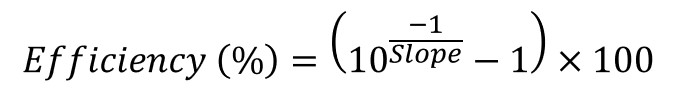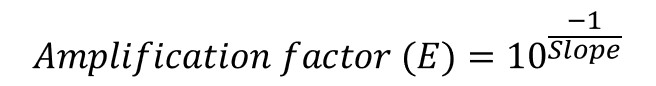qPCR Primer Efficiency Calculator

Below is a PCR primer efficiency calculator. All that is needed for the calculator is the slope of the standard curve during the serial dilutions during the qPCR. Once entered, hit the calculate button and the primer efficiency (as a percentage) and the amplification factor (E) will be returned.

Mastering qPCR

A video tutorial on qPCR primer efficiency calculation can be found in our Mastering qPCR course.
>>Use code 20QPCR to get 20% off<<

How to use the calculator

1. Calculate the slope of the curve during the primer efficiency qPCR. If you are struggling to determine the slope of the curve, check out our guide on how to calculate primer efficiencies in PCR.

2. Click the calculate button.

3. The calculator will determine and return the amplification factor and primer efficiency.

Note: the slope should be a negative value. For example, a slope of -3.32 will produce a primer efficiency of 100% and an amplification factor of 2.

How the calculator works

Primer efficiency (%)

To determine the primer efficiency as a percentage from the slope, this is calculated as follows:Amplification factor (E)

The amplification factor (E) is calculated using the following equation:1.nazanin
•Dr Steven Bradburn, PhD